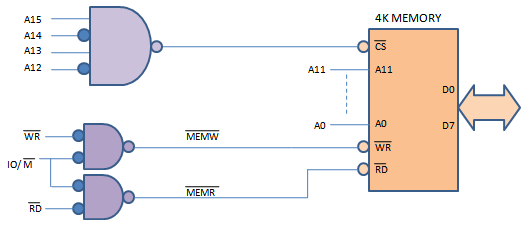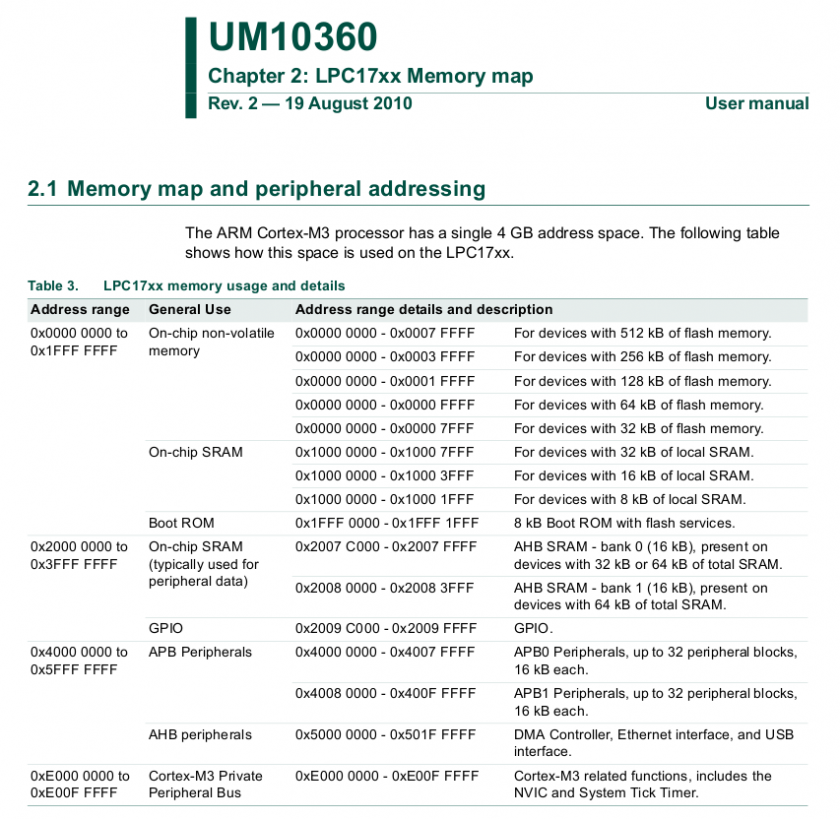GPIO

Learn how to manipulate and read the voltage levels of the pins on the SJ Board.

Bit Manipulation

Bit-masking is a technique to selectively modify individual bits without affecting other bits.

Bit SET

To set a bit, we need to use the OR operator. This is just like an OR logical gate you should've learned in your Digital Design course.

// We want to set Bit #7 of a variable called: REG
REG = REG | 0x80;
// Let's set bit #31:
REG = REG | 0x80000000;
// Here is an easier way to write these:
// (1 << 31) means 1 gets shifted left 31 times to produce 0x80000000
REG = REG | (1 << 31);
// Simplify further:
REG |= (1 << 31);
// Set Bit #21 and Bit #23 at the same time
REG |= (1 << 21) | (1 << 23);

Bit CLEAR

To set a bit to 0, in other words reset or clear a bit, the logic is similar, but instead of ORing a bit, we will an AND function to clear. Note: that ANDing something with 0 clears it and ANDing something with a 1 does not change it. The tilde (~) operator can help us invert the bits of a value in the following examples:

// Assume we want to reset Bit#7 of a register called: REG
REG = REG &   0x7F;
REG = REG & ~(0x80); // Same thing as above, but using ~ is easier

// Let's reset bit#31:
REG = REG & ~(0x80000000);

// Let's show you the easier way:
REG = REG & ~(1 << 31);

// Simplify further:
REG &= ~(1 << 31);

// Reset Bit#21 and Bit# 23:
REG &= ~( (1 << 21) | (1 << 23) );

Bit TOGGLE

// Using XOR operator to toggle 5th bit
REG ^= (1 << 5);

Bit CHECK

Suppose you want to check bit 7 of a register is set:

bool check_bit = REG & (1 << 7);
if(check_bit)
{
DoAThing();
}

Now let's work through another example in which we want to wait until bit#9 is 0:

// One way:
while(REG & (1 << 9) != 0)
{
continue;
}
// Another way:
while(REG & (1 << 9))
{
continue;
}

Multi-Bit Insertion

// Insert a set of continguous bits into a target value.
// Value within target is unknown. This is shown using X's
//
// target   =        0xXXXX'XXXX
//                        ^
//                       /
//                      /
// value   = 0xABCD --+
// position = 16
// width    = 16
//
// return   =        0xABCD'XXXX

// First you must clear the bits in that location
target &= ~(0xFFFF << 16);
// Now that there are only 0s from position 16 to 31, ew
// can OR those bits with our own set of 1s.
target |=  (0xABCD << 16);

Multi-Bit Extraction

/// Extract a set of contiguous bits from a target value.
///
/// target   =        0x00FE'DCBA
///                            ^
///                           /
///                          /
/// value  = 4 -----------+
/// width    = 8
///
/// return   = 0xCB

// Shift target to the left by 4 to make the 0th bit the start of the bits you want to extract.
// Store the result in to a local variable
uint32_t result = target >> 4;
// Since we only want 8 bits from the result, we need to clear away the rest of the bits from
// the original target.
// AND the result with 0xFF, to clear everything except for the first 8 bits.
result = result & 0xFF;

LPC40xx Memory Map

What is a Memory Map

memory map is a layout of how the memory maps to some set of information. With respect to embedded systems, the memory map we are concerned about maps out where the Flash (ROM), peripherals, interrupt vector table, SRAM, etc are located in address space.

Memory mapped IO

Memory mapped IO is a a means of mapping memory address space to devices external (IO) to the CPU, that is not memory.

For example (assuming a 32-bit system)
• Flash could be mapped to addresses 0x00000000 to 0x00100000 (1 Mbyte range)
• GPIO port could be located at address 0x1000000 (1 byte)
• Interrupt vector table could start from 0xFFFFFFFF and run backwards through the memory space
• SRAM gets the rest of the usable space (provided you have enough SRAM to fill that area)

It all depends on the CPU and the system designed around it.

Port Mapped IO

Port mapped IO uses additional signals from the CPU to qualify which signals are for memory and which are for IO. On Intel products, there is a (~M/IO) pin that is LOW when selecting MEMORY and HIGH when it is selecting IO.

The neat thing about using port mapped IO, is that you don't need to sacrifice memory space for IO, nor do you need to decode all 32-address lines. You can limit yourself to just using 8-bits of address space, which limits you to 256 device addresses, but that may be more than enough for your purposes.Figure 2. Address Decoding with port map

(http://www.dgtal-sysworld.co.in/2012/04/memory-intercaing-to-8085.html)

LPC40xx memory mapFigure 3. LPC17xx Memory Map, which is nearly the same as the LPC40xx memory map

From this you can get an idea of which section of memory space is used for what. This can be found in the UM10562 LPC40xx user manual. If you take a closer look you will see that very little of the address space is actually taken up. With up to 4 billion+ address spaces (because 2^32 is a big number) to use you have a lot of free space to spread out your IO and peripherals.

Reducing the number of lines needed to decode IO

The LPC40xx chips, reduce bus line count, make all of the peripherals 32-bit aligned. Which means you must grab 4-bytes at a time. You cannot grab a single byte (8-bits) or a half-byte (16-bits) from memory. This eliminates the 2 least significant bits of address space.

Accessing IO using Memory Map in C

Please read the following code snippet. This is runnable on your system now. Just copy and paste it into your main.cpp file.

//The goal of this software is to set the GPIO pin P1.0 to
// low then high after some time. Pin P1.0 is connected to an LED.
// The address to set the direction for GPIOs in port 1 is below:
//
//     FIO1DIR = 0x2009C020
//
//  The address to set the output value of a pin in port 1 is below:
//
//     FIO1PIN = 0x2009C034

#include <cstdint>

volatile uint32_t * const FIO1DIR = (uint32_t *)(0x2009C020);
volatile uint32_t * const FIO1PIN = (uint32_t *)(0x2009C034);

int main(void)
{
// Set 0th bit, setting Pin 0.0 to an output pin
*FIO1DIR |= (1 << 0);
// Set 0th bit, setting Pin 0.0 to high
*FIO1PIN &= ~(1 << 0);
// Loop for a while (volatile is needed, otherwise this will not loop for very long!)
for(volatile uint32_t i = 0; i < 1000000; i++);
// Clear 0th bit, setting Pin 0.0 to low
*FIO1PIN |= (1 << 0);
return 0;
}

volatile keyword tells the compiler not to optimize this variable out, even if it seems useless

const keyword tells the compiler that this variable cannot be modified

Notice "const" placement and how it is placed after the uint32_t *. This is because we want to make sure the pointer address never changes and remains constant, but the value that it references should be modifiable.

Using the LPC40xx.h

The above is nice and it works, but its a lot of work. You have to go back to the user manual to see which addresses are for what register. There must be some better way!!

Take a look at the LPC40xx.h file, which It is located in the SJSU-Dev/firmware/library/L0_LowLevel/LPC40xx.h. Here you will find definitions for each peripheral memory address in the system.

Lets say you wanted to port the above code to something a bit more structured:

• Open up "LPC40xx.h
• Search for "GPIO"
• You will find a struct with the name LPC_GPIO_TypeDef.
• Now search for "LPC_GPIO_TypeDef" with a #define in the same line.
• You will see that LPC_GPIO_TypeDef is a pointer of these structs
• #define LPC_GPIO0 ((LPC_GPIO_TypeDef *) LPC_GPIO0_BASE )
• #define LPC_GPIO1 ((LPC_GPIO_TypeDef *) LPC_GPIO1_BASE )
• #define LPC_GPIO2 ((LPC_GPIO_TypeDef *) LPC_GPIO2_BASE )
• #define LPC_GPIO3 ((LPC_GPIO_TypeDef *) LPC_GPIO3_BASE )
• #define LPC_GPIO4 ((LPC_GPIO_TypeDef *) LPC_GPIO4_BASE )
• We want to use LPC_GPIO1 since that corrisponds to GPIO port 1.
• If you inspect LPC_GPIO_TypeDef, you can see the members that represent register FIODIR and FIOPIN
• You can now access FIODIR and FIOPIN in the following way:
#include "LPC40xx.h"

int main(void)
{
// Set direction of P0.0 to 1, which means OUTPUT
LPC_GPIO1->FIODIR |= (1 << 0);
// Set 0th bit, setting Pin 0.0 to high
LPC_GPIO1->FIOPIN &= ~(1 << 0);
for(volatile uint32_t i = 0; i < 1000000; i++);
// Clear 0th bit, setting Pin 0.0 to low
LPC_GPIO1->FIOPIN |= (1 << 0);
return 0;
}

At first this may get tedious, but once you get more experience, you won't open the LPC40xx.h file very often. This is the preferred way to access registers in this course.

On occasions, the names of registers in the user manual are not exactly the same in this file.

General Purpose Input Output

Objective

To be able to General Purpose Input Output (GPIO), to generate digital output signals and to read input signals. Digital outputs can be used as control signals to other hardware, to transmit information, to signal another computer/controller, to activate a switch or, with sufficient current, to turn on or off LEDs or to make a buzzer sound.

Below will be a discussion on using GPIO to drive an LED.

Although the interface may seem simple, you do need to consider hardware design and know some of the fundamental of electricity. There are a couple of goals for us:

• No hardware damage if faulty firmware is written.
• Circuit should prevent excess amount of current to avoid processor damage.